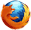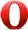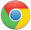# JavaScript POSITIVE_INFINITY 属性JavaScript Number 对象

## 实例

Number.POSITIVE_INFINITY;

Infinity

## 定义和用法

POSITIVE_INFINITY 属性值代表正无穷大。

POSITIVE_INFINITY 属性表示大于 Number.MAX_VALUE 的值。

## 使用 Number.POSITIVE_INFINITY

POSITIVE_INFINITY 是 JavaScript Number 对象的静态方法。

## 实例

var x = 100;
x.NEGATIVE_INFINITY;

x 输出结果:

undefined

## 浏览器支持## 语法

Number.POSITIVE_INFINITY;

## 技术细节

返回值： The numeric value: Infinity 1.1

## 实例

var n=(Number.MAX_VALUE)*2;

n 输出结果:

InfinityJavaScript Number 对象